# Striking a Balance: Exploring Fairness in Buffer Allocation and Packet Scheduling

Recently, I’ve been contemplating the concept of fairness, and I see interesting parallels between being a parent and being a network professional. As human beings, we have an inherent, intuitive sense of fairness that manifests itself in various everyday situations. Let me illustrate this idea with a couple of hypothetical scenarios:

Scenario 1: Imagine I’m a parent with four young children, and I’ve ordered a pizza for them to share. If I want to divide the pizza fairly among the children, fairness would mean that each child receives an equal portion - in this case, one-quarter of the pizza.

Scenario 2: Now let’s say I’ve ordered another pizza for the same four children, but one of the kids only cares for pizza a little and will only eat one-tenth of his share. In this situation, it wouldn’t be fair for me to give that child who doesn’t like pizza more than one-tenth of the piece because the excess would go to waste. The fair way to divide the pizza would be to give the child who doesn’t like pizza a one-tenth portion and split the remaining nine-tenths evenly among the other three kids.

The approach mentioned in the second scenario represents a principle of fairness known as “max-min fairness,” where you allocate resources starting with the least demanding user, then the following least demanding user, and so on.

More broadly, some overarching goals of fairness in the context of networking include:

• Distributing available resources in an equitable manner
• Isolating badly-behaved users who abuse the system
• Achieving statistical multiplexing, meaning links are divided efficiently.
• Allowing a single user flow to utilize capacity if no competing flows exist.
• Using a work-conserving scheduler that never leaves a link idle if packets are waiting to be sent.

# Max-Min Fairness

The idea behind max-min fairness is prioritizing smaller demands before allocating excess capacity to larger demands. Specifically, a max-min fair allocation follows these principles:

1. First, fulfill the smallest demand.
2. Once the smallest demand is met, fulfill the subsequent smallest demand.
3. Continue satisfying demands from smallest to largest until all demands are met, or resources are exhausted.
4. If resources remain after all demands are satisfied, allocate the excess to demands fairly.

A common algorithm for achieving max-min fairness operates iteratively:

1. Calculate the fair share for each unsatisfied user.
2. Allocate this fair share to each user.
3. Reclaim any over-allocations and save them as residual capacity.
4. Recalculate fair shares and allocate residual capacity to remaining unsatisfied users.
5. Repeat steps 2-4 until all users are satisfied or resources are fully allocated.

By prioritizing small demands first, max-min fairness aims to maximize fairness for users with varying needs. It ensures resources are divided equitably rather than simply proportionally. The step-wise iterative approach satisfies demands in order from smallest to largest.

Example of Max-Min Fair Bandwidth Assignment

Let’s look at an example with a bottleneck link with 10 Gbps of bandwidth shared by four flows. The total demand from the four flows exceeds 10 Gbps. To allocate the 10 Gbps fairly, we’ll go through three iterative rounds; in each round, we allocate the residual bandwidth to the unsatisfied flows.

Iteration1: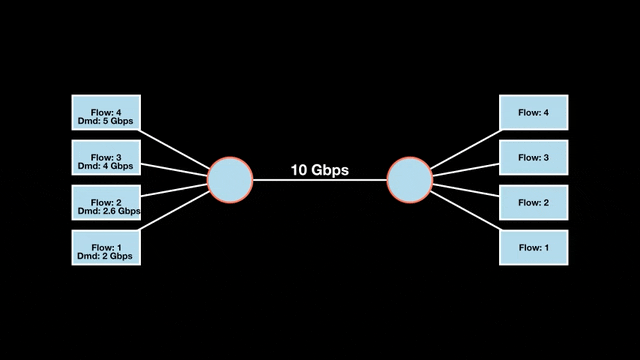Iteration2: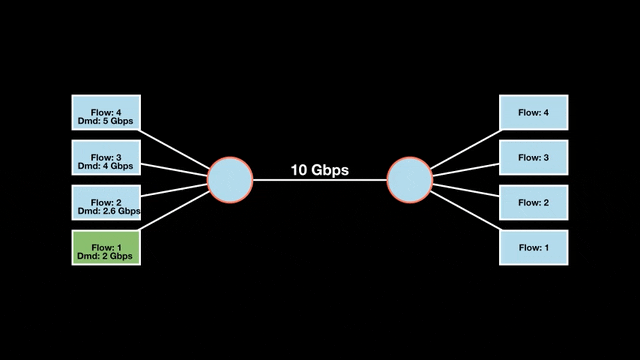Iteration3: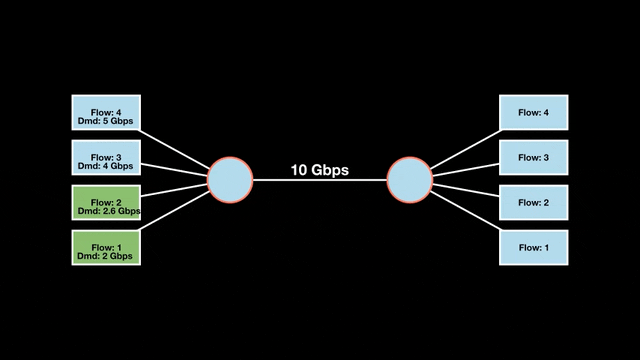Max-min fairness maximizes smaller demands first until resources are exhausted. Flows receive their full demanded bandwidth if possible or the maximum allocation if resources are insufficient, as for Flows 3 and 4. The goal is to prioritize fairness for users with lower demands.

Note that “maximizing” a flow means giving it the highest possible bandwidth allocation according to what remains available. It does not mean fully satisfying each flow’s total demand.

Weighted Max-Min Fairness

Max-min fairness can be extended by assigning weights to demands. Treat a demand of x units with weight w as w separate demands of x/w units each. An allocation is weighted max-min fair if it satisfies max-min fairness for these weighted demands. The algorithm is similar:

1. Calculate fair share as available resources / total weight.
2. Allocate one fair share unit to each unit of weight.
3. Reclaim any over-allocations.
4. Recalculate and allocate residual to remaining unsatisfied demands.
5. Repeat until resources are fully allocated.

This extends max-min fairness by considering demands and weights. It allows a more balanced distribution of resources across varying demands.

Example of Weighted Max-Min Fair Bandwidth Assignment

In the below example, we iterate in 2 rounds till no residual bandwidth is left. In step 1, we sum the weights and divide the resource to get the fair share unit per weight and then allocate to the flows.

Iteration 1: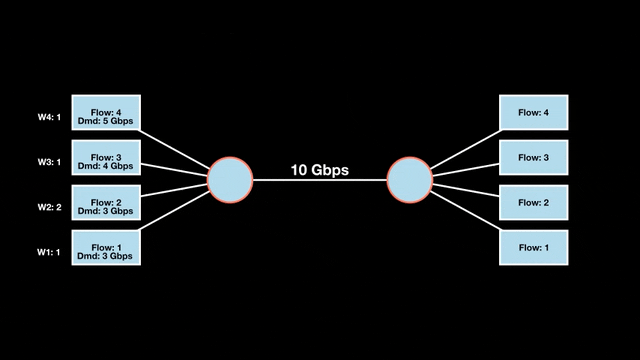Iteration 2: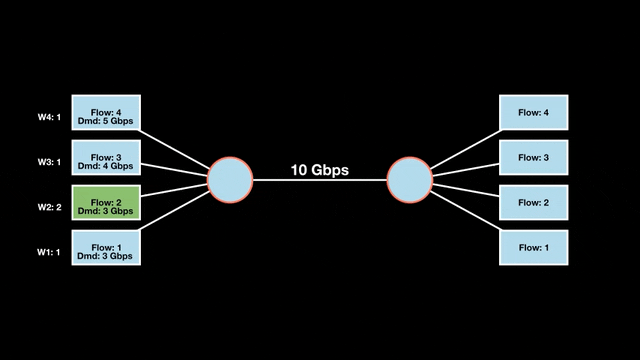## Implementation Challenges

Unlike the fixed example of kids sharing pizza, network flows are dynamic, constantly changing as flows come and go. This dynamic nature makes it impractical to implement exact max-min fairness.

Instead, a more practical approach is to consider the degree of unfairness, which measures the difference in service received between flows over time. For instance, let’s denote the service received by flow “a” in a time interval $[t_{1}, t_{2}]$ as $S_{a}(t_{1}, t_{2})$, and the service received by flow “b” in the same interval as $S_{b}(t_{1}, t_{2})$. The degree of unfairness is then given by the difference between these service values, expressed as $|S_{a}(t_{1}, t_{2}) - S_{b}(t_{1}, t_{2})|$.

A fair method does not favor any specific flow, while an unfair method tends to favor certain flows over others, resulting in some flows receiving more service over time. As time progresses, the time interval $[t_{1}, t_{2}]$ increases, and the difference in the received service also increases, i.e., $|S_{a}(t_{1}, t_{2}) - S_{b}(t_{1}, t_{2})|$.

To determine fairness, we expect that the absolute difference between the received services of flow “a” and flow “b” in any time interval should be less than or equal to some constant, denoted as $c$. In other words, $|S_{a}(t_{1}, t_{2}) - S_{b}(t_{1}, t_{2})| \le c$ for every interval.

In the case of weighted fairness, we first normalize the services and then compare the difference in the normalized values, which should also be less than the constant $c$. The normalized services can be expressed as $\frac{S_{a}(t_{1}, t_{2})}{r_{a}}$ and $\frac{S_{b}(t_{1}, t_{2})}{r_{b}}$, where $r_{a}$ and $r_{b}$ are normalization factors for flow “a” and flow “b,” respectively.

So, the condition for weighted fairness can be stated as $|\frac{S_{a}(t_{1}, t_{2})}{r_{a}} - \frac{S_{b}(t_{1}, t_{2})}{r_{b}}| \le c$. The smaller the difference between the normalized services, the more fair the method is, and if the difference is 0, then the method is perfectly fair.

# Buffer Management

## Background

Before diving into the details, let’s get a high-level understanding of the architecture involved. In most network platforms, buffering is primarily implemented at the ingress side (ignoring smaller egress buffers). The ingress Traffic Manager component is responsible for packet queuing and scheduling. These queues at the ingress side represent the packet queues on the output port, forming what are known as Virtual Output Queues (VOQs). When packets arrive at the ingress, they are enqueued in these VOQs and await a VOQ grant message to proceed to the egress packet processor.

The number of VOQ queues on the ingress side is determined by the total number of egress ports multiplied by the number of queues per egress port. Traffic is classified and mapped to these queues and then scheduled to the egress output queues.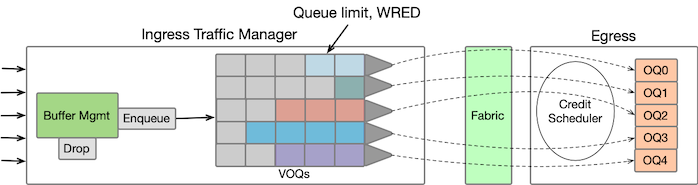The buffer memory is organized into fixed cell sizes. If a packet is larger than the cell size, it occupies multiple cells plus some overhead for each packet. Regarding buffer allocation, a portion of the buffer memory is reserved for each queue, while the remaining space is shared across all the queues, with allocation occurring on demand. When a packet arrives, the system first checks the reserved pool of the corresponding queue to determine if there is space available. If there is sufficient space, the packet is enqueued in that queue. However, if the reserved pool of the queue is full, the traffic manager looks at the shared pool for buffer space allocation.

A Dynamic Threshold algorithm is employed to make the shared pool buffer space allocation efficient and fair. This algorithm dynamically sets the threshold for each queue based on the buffer utilization. If a queue’s buffer utilization exceeds its dynamic threshold, the incoming packet is dropped to prevent excessive congestion. On the other hand, if the buffer utilization is below the threshold, the shared pool memory is allocated to that queue.

The concept behind the shared pool is to utilize the buffer memory effectively by leveraging the statistical multiplexing nature of the traffic. This way, the buffer resources are distributed fairly among the active queues, ensuring efficient utilization and congestion control.

## Dynamic Threshold Workings

So, the question that arises now is: how does this dynamic threshold algorithm actually work? In many cases, these details may not be publicly disclosed and may be protected by Non-Disclosure Agreements (NDA). However, for our purposes, we will primarily refer to the work published in “Dynamic Queue Length Thresholds for Shared-Memory Packet Switches” which I believe is the most commonly deployed algorithm. Once we understand this then it becomes easier to explore how other implementation approaches tackle similar challenges and enables us to ask the right questions.

### Static Threshold (ST) and Push Out(PO) Buffer management schemes

#### Static Threshold

One approach to buffer allocation involves setting static thresholds per queue and determining the maximum queue length. Cells are accepted if the queue length is below the threshold but dropped if it exceeds the threshold. The challenge with this method is that thresholds remain fixed and don’t adapt to changing traffic loads. If thresholds are too low, queues may discard cells excessively, even with available buffer space. Conversely, if thresholds are too high, some queues may monopolize buffer space, causing starvation for others. Careful tuning and adjustments are required to optimize buffer management based on expected traffic loads, making it less ideal for network operation.

#### Push Out

With Push-Out (PO), an incoming cell can enter a full buffer by displacing another cell. Specifically, the new cell pushes out the cell at the head of the longest queue. The pushed cell loses its buffer space, but the new cell does not take the exact same position. Instead, it joins its own queue. This lets smaller queues grow by reclaiming space from longer queues.

Key benefits of PO:

• Ensures fairness - shorter queues grow by pushing out longer queues
• Maximizes efficiency - no starving or idle buffer space
• Adaptive - queue lengths self-regulate based on activity
• High overall throughput

However, PO has some implementation challenges:

• Writing new cells requires extra push-out steps, potentially slowing performance.
• Need to monitor all queue lengths and identify the longest queue.
• Complex for multiple priority traffic classes.
• Overheads to remove and repair pushed-out low-priority cells.

In summary, while PO provides excellent fairness and utilization, its advantages come at the cost of extra complexity. The overheads of push-out operations and length monitoring should be evaluated when considering PO for a specific application.

### Dynamic Threshold

In the case of the DT (Dynamic Threshold) scheme, the threshold or maximum queue length is dynamically adjusted based on the unused buffer space. Specifically, the threshold is set to $\alpha$ times the remaining buffer space, where $\alpha$ is a configurable proportionality constant.

$\hspace{3cm} T(t) = \alpha*Unused\:Buffer\:Space = \alpha*(B - Q(t)) \tag{eq.1} \\$

where, $T(t)$ is the dynamic queue threshold of port. $\alpha$ is the power of two (positive or negative) $B$ is the total Buffer space. $Q(t)$ Total buffer occupied at time t. Sum of all Queue lengths.

In steady state, Total buffer occupancy can be given by $Q = S\ast T + \Omega$ where $S$ is the active queues which are hitting the threshold $T$ and $\Omega$ is the space occupied by the uncontrolled queues which are below the threshold (uncontrolled queues). Substituting this in $eq.1$ we can find the steady state length $Q^{i}$ of each controlled queue.

$\hspace{3cm} T = \alpha * (B - (S*T+\Omega)) \\$ $\hspace{3cm} T = \alpha * B - \alpha*S*T - \alpha * \Omega \\$ $\hspace{3cm} T+\alpha*S*T = \alpha*B-\alpha*\Omega \\$ $\hspace{3cm} T(1+\alpha*S) = \alpha*(B-\Omega) \\$ $\hspace{3cm} T = \frac{\alpha*(B-\Omega)}{1+\alpha*S} = Q^{i} \tag{eq.2} \\$

When the buffer utilization is low, the thresholds will be higher, allowing longer queues. Conversely, when the buffer space becomes scarce, the thresholds will be lower to limit queue lengths. This adaptive threshold computation only requires knowledge of the total buffer occupancy and eliminates the need for per-queue monitoring. Implementing $\alpha$ can be easily achieved using a shift register, especially when the values are powers of two, such as 1/16, 1/8, 2, 8, 16, etc.

An important aspect of the DT approach is that it intentionally maintains a small reserve of buffer space that is not allocated. The remaining non-reserved space is distributed equally among all the active queues. This strategic reserve prevents issues related to starvation and unfairness that might arise with static thresholds.

For example, with $\alpha=2$, the algorithm aims to maintain each queue’s length at approximately twice the spare buffer capacity. If there is only one active queue, denoted as $S=1$, then using equation (2), the active queue will be allocated $\frac{2}{1+2 \times 1} \times \text{BufferSpace}$, which amounts to 2/3 of the available buffer space, while the remaining 1/3 is kept reserved and unallocated.

In the case of two active queues, each queue will receive $\frac{2}{1+2 \times 2} \times \text{BufferSpace}$, which equals 2/5 of the free buffer space for each queue. The remaining 1/5 is reserved and remains unallocated.

The key observation is that as the number of active queues increases, the amount of reserved space decreases. This dynamic behavior ensures that as more queues become active, each queue receives a fair share of the available buffer space, promoting efficient buffer utilization and preventing excessive wastage of resources.

## Exploring Buffer Occupancy and Dynamic Threshold through Simulations

Let’s delve into the topic through simple discrete event-based simulations. While these simulations may not capture all real-life complexities, they serve to build our intuition. As George Box, the renowned statistician, once said, “All models are wrong, but some are useful.”

### Buffer Occupancy without Dynamic Threshold

In this scenario, we have a buffer size of 100 cells, and each packet consumes a single cell. Four queues are of equal priority, and packets for each queue are generated using a Poisson distribution, resulting in each queue getting between 0 to 6 packets in each cycle.

In each simulation iteration, packets are added to the buffer if the buffer is not full, i.e., buffer occupancy is less than 100. Then, in the same iteration, one packet is removed from each queue in a round-robin fashion. Since the buffer drain rate is slower than the arrival rate, we can expect the queues to become backlogged. Once the buffer reaches its full capacity, packets start getting dropped.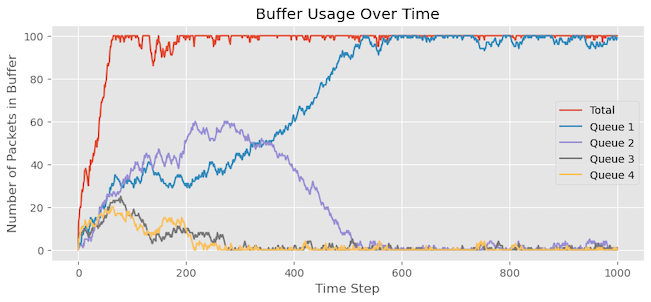Total packets generated: 4943
Total packets dropped: 2145
Queue1: Total packets generated 1255,dropped 157,pct_dropped 12.51%
Queue2: Total packets generated 1235,dropped 466,pct_dropped 37.73%
Queue3: Total packets generated 1176,dropped 649,pct_dropped 55.19%
Queue4: Total packets generated 1277,dropped 873,pct_dropped 68.36%


The simulation plot reveals several key observations:

1. The buffer utilization reaches its maximum capacity, causing packet drops once it hits the limit.
2. Among the queues, Queue 1 has the highest number of packets in the buffer, while Queues 2, 3, and 4 seem to be experiencing starvation with limited access to the buffer.
3. The statistics at the end of the simulation confirm this observation, showing that Queue 3 and Queue 4 experienced the highest number of packet drops.

This outcome is due to the round-robin approach for adding packets to the buffer. Queue 1 consistently receives priority and consumes the available resources first. As a result, it starves the other queues, leading to fewer packets from Queues 2, 3, and 4 entering the buffer and facing more packet drops.

Because of the randomness involved, we may have observed this just by luck. To ensure our observations’ robustness, we repeated the experiment 100 times and analyzed the mean percentage of packet drops for each Queue. As depicted in the plot below, the results consistently show that Queues 2, 3, and 4 are indeed experiencing starvation, leading to a higher percentage of packet drops than Queue 1.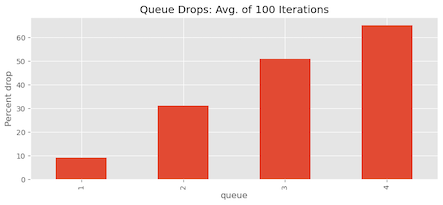### Buffer Occupancy with Dynamic Threshold

Let’s replicate the experiment with dynamic threshold. We will add this condition to our code which checks if the current queue length is less than the $\alpha \times (\text{buffersize} - \text{buffer_occupied}))$ with $\alpha=2$.

if self.queue_packets[packet.source] <
packet.alpha * (self.limit - len(self.packets)):


The plot below showcases the results of our simulation, and we can derive several observations from it:

1. In contrast to the previous experiment, the buffer utilization does not reach its maximum capacity. This behavior is associated with the dynamic threshold mechanism, which deliberately reserves some buffer space.
2. We previously discussed the steady-state queue allocation formula: $\frac{\alpha}{1+\alpha \times S} \times \text{BufferSpace}$. With four active queues, each queue is allocated approximately $\frac{2}{9}$ of the buffer space (around 22 cells), while $\frac{1}{9}$ i.e., 11 cells are reserved from the 100 cells available. Thus, the maximum expected utilization is approximately 89 cells.
3. It is evident that Queues 2, 3, and 4 are not experiencing starvation as they did in the previous experiment. Most queues maintain a state of equilibrium with the resource allocation.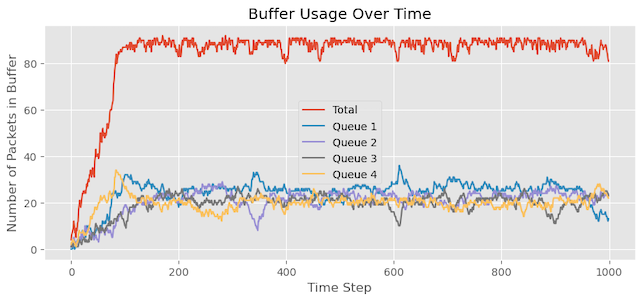Total packets generated: 4831
Total packets dropped: 759
Queue1: Total packets generated 1250,dropped 243,pct_dropped 19.44%
Queue2: Total packets generated 1150,dropped 128,pct_dropped 11.13%
Queue3: Total packets generated 1183,dropped 161,pct_dropped 13.61%
Queue4: Total packets generated 1248,dropped 227,pct_dropped 18.19%


Once again, to ensure the reliability of our findings, we repeat the experiment 100 times and observe the mean percentage of packet drops for each Queue. As shown in the plot below, it’s evident that the Queue allocation is now much fairer, and no Queue is experiencing starvation and the buffer drops for each queue is similar.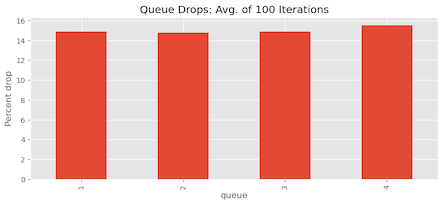### Buffer Occupancy with Dynamic Threshold and WRR

In this scenario, we introduce a priority scheme to prioritize Queue 4 over the other queues. Initially, each queue had a priority of 1, but now we will increase the priority of Queue 4 to 2. This change means that twice the number of packets will be drained from the buffer for Queue 4 in each cycle compared to other queues. As a result, the buffer occupancy for Queue 4 packets will be lower than the other queues.

It’s important to clarify that high weights in this context do not determine the priority to access the buffer initially. Instead, the weights define the order in which packets are drained from the buffer for the already present packets.

The plot below illustrates the output of a single run, aligning with our expectations. Queue 4 exhibits minimal buffer occupancy, and the number of packet drops for Queue 4 is zero, as the statistics below indicate. Additionally, the remaining queues, all of equal priority, share similar buffer occupancy.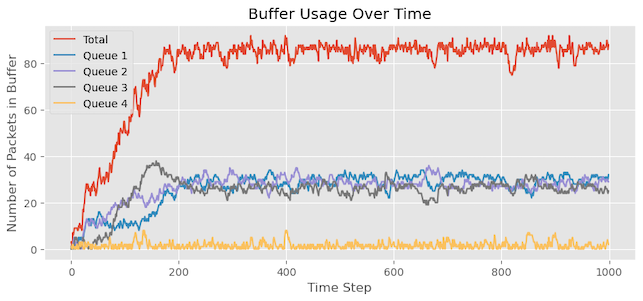Total packets generated: 4709
Total packets dropped: 459
Total packets dropped from Queue 1: 128
Total packets dropped from Queue 2: 164
Total packets dropped from Queue 3: 167
Total packets dropped from Queue 4: 0
Total packets added to buffer: 4250


By repeating the experiment 100 times and analyzing the mean results, we observe that Queue 4 experiences no packet drops.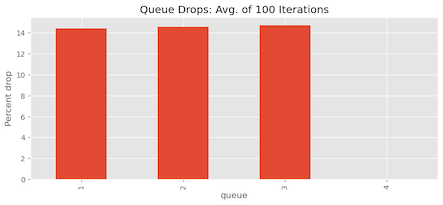### Changing the Traffic assumptions

Now, we will investigate the impact of traffic with a more bursty nature. To achieve this, we will modify the previous assumption of Poisson traffic and instead use the Batch Markovian Arrival Process (BMAP) to generate packets. BMAP generates packets that exhibit more bursty behavior than packet arrivals in Poisson traffic.

The plot below shows the difference in the number of packets arriving for each time unit between Poisson traffic and BMAP traffic scenarios. In the case of Poisson traffic, the packet arrivals are more consistent and evenly spread over time. Conversely, with BMAP traffic, packet arrivals are less frequent. Still, when they occur, they are more bursty, resulting in periods of low packet arrivals followed by sudden bursts of multiple packets arriving quickly.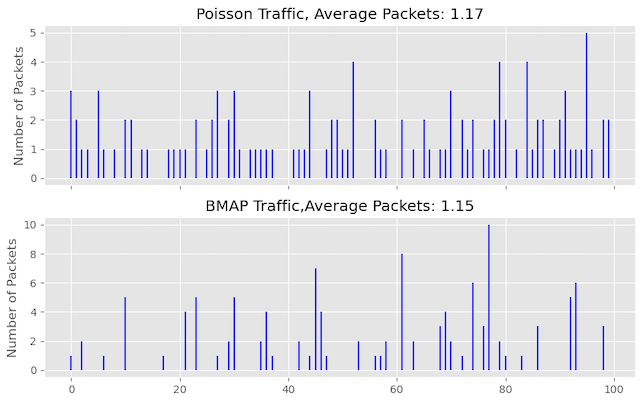### Buffer Occupancy with Dynamic Threshold and WRR for bursty traffic

In this scenario, I have made the traffic more bursty in nature while maintaining Queue 4’s higher weight (weight = 2) compared to other queues. Comparing the graph with the previous experiment, we can observe that more packets for Queue 4 are staying in the buffer. We also notice that Queue 4 experiences now some packet drops which we didn’t see earlier.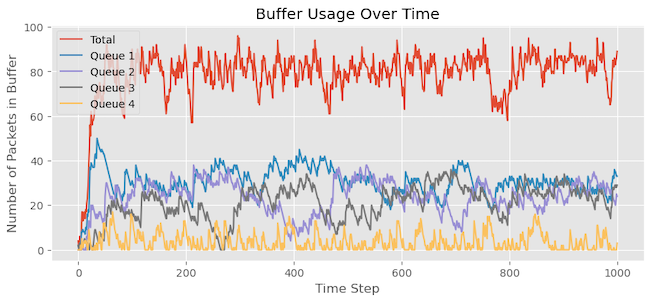Total packets generated: 4990
Total packets dropped: 631
Total packets dropped from Queue 1: 257
Total packets dropped from Queue 2: 145
Total packets dropped from Queue 3: 211
Total packets dropped from Queue 4: 18
Total packets added to buffer: 4359


Repeating the experiment multiple times and analyzing the mean results, we find that on average, there are 1.37% packet drops for Queue 4:

queue drops
1    19.95%
2    12.54%
3    17.18%
4     1.37%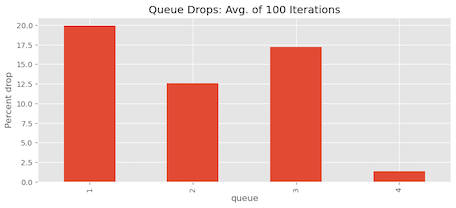To address the packet loss issue for Queue 4, an approach we can take is increasing the alpha value from 2 to 4. By doing so, Queue 4 will be allocated a larger portion of the buffer compared to the other queues. The aim is to determine if this adjustment reduces or eliminates the packet drops for Queue 4. However, upon analyzing the statistics, we observe that although the packet drops have decreased, they have not been completely eliminated.

Total packets generated: 4981
Total packets dropped: 637
Total packets dropped from Queue 1: 57
Total packets dropped from Queue 2: 134
Total packets dropped from Queue 3: 432
Total packets dropped from Queue 4: 14
Total packets added to buffer: 4344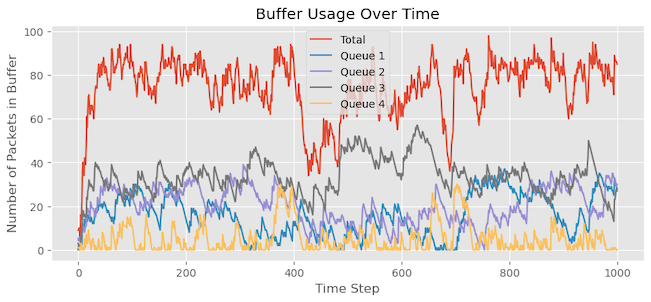The below results show the impact of different alpha values of 4, 8, and 16. The purpose was to observe how adjusting the alpha value influences packet drops in the system. The findings revealed that increasing the alpha value from 4 to 8 and 16 did improve packet drop reduction but did not remove them altogether.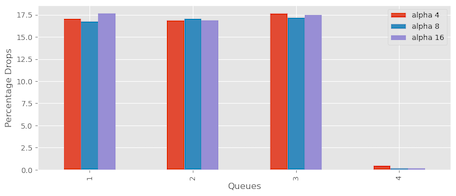#### Let’s summarize our progress so far:

• We have explored the concept of the Dynamic Threshold scheme for buffer management, understanding how it dynamically adjusts buffer sharing based on the unused buffer capacity. The threshold value changes adaptively according to the available buffer space.
• As confirmed by our simulations, the Dynamic Threshold scheme strategically reserves a small buffer space and distributes the remaining buffer equally among the active queues.
• Through comparisons between scenarios with and without the Dynamic Threshold, we have observed how it enhances fairness in buffer allocation among active queues and effectively prevents starvation.
• We have also studied buffer draining using Weighted Round-Robin (WRR) and its impact on buffer occupancy.
• To assess the scheme’s performance under bursty traffic conditions, we introduced more bursty traffic using the Batch Markovian Arrival Process (BMAP). We observed that increasing the alpha value improved the loss percentage but we were not able to get rid of the packet loss completely.

It’s important to highlight that we wholly ignored the two key factors here, the packet size distribution and the congestion control backoff behavior when they observe packet loss. This was because the amount of code I had to write was much more effort than the time I had.

## Round Robin and Deficit Round Robin: Ensuring Fairness in Buffer Draining

In the context of buffer draining, we have employed both round-robin and weighted round-robin scheduling techniques. While the basic round-robin approach can achieve fairness when all queues have equal weights and uniform packet sizes, it will lead to imbalances when the queues contain packets of varying lengths. For instance, if one queue primarily holds 500-byte packets while another mainly contains 1500-byte packets, the round-robin approach may result in unequal shares for the queues, despite having equal priority.

To address this challenge, the deficit round-robin (DRR) scheduling algorithm, proposed by Sheerhar and VargheseEfficient Fair Queuing Using Deficit Round-Robin, comes to the rescue. DRR ensures fairness even when the packets have different sizes. It achieves this by introducing a “deficit counter” representing the number of bytes a queue can transmit when its turns come. Additionally, it maintains a “quantum” counter, which specifies the number of bytes added to the deficit counter of a queue at each round. You can think of the quantum as a credit accumulating with each iteration.

The operation of a DRR scheduler can be summarized as follows:

1. Packets from queues are transmitted in a round-robin fashion, with the deficit counter initialized to zero.
2. Before servicing a flow, the quantum value is added to the deficit counter of that flow: deficit counter += quantum.
3. A packet from a queue is only transmitted if the deficit counter is greater than or equal to the length of the packet: deficit counter >= packet length.
4. If a packet is transmitted, the deficit counter of that flow is updated by subtracting the number of bytes in the transmitted packet: deficit counter -= #bytes in the packet.

By incorporating the deficit round-robin algorithm, we can ensure fairness in buffer draining, even when the packets have varying sizes across different queues. This approach promotes more efficient and equitable utilization of network resources.

As an example, consider two iterations with a quantum size of 1000 bytes:

First Iteration:

• A’s count = 1000 (No packet is served since 1500 > 1000 deficit counter)
• B’s count = 200 (both packets are served.)
• count = 1000 (No packet is served since 1200 > 1000 deficit counter)

Second Iteration:

• A’s count = 500 (Packet is served: 2000 - 1500 = 500)
• B’s count = 0
• C’s count = 800 (Packet is served: 2000 - 1200 = 800)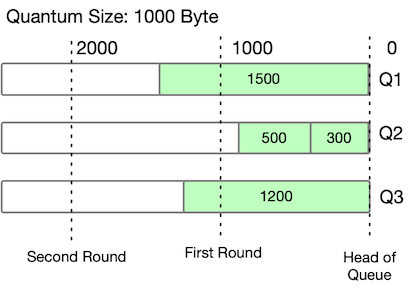We can also compare the schemes using discrete event simulation. We consider two flows: Flow 0 and Flow 1. Flow 0 generates packets ranging from 500 to 1500 bytes, while Flow 1 generates packets ranging from 100 to 500 bytes. Both flows are directed to two different scheduling algorithms: Weighted Round-Robin (WRR) and Deficit Round-Robin (DRR) schedulers.

For the DRR scheduler, we have set the quantum size to 1500 bytes. The simulation captures the arrival of packets from both flows at the packet sink side under these scheduling schemes. By analyzing the simulation results, we can observe how the packets from each flow are processed and drained using WRR and DRR schedulers, respectively.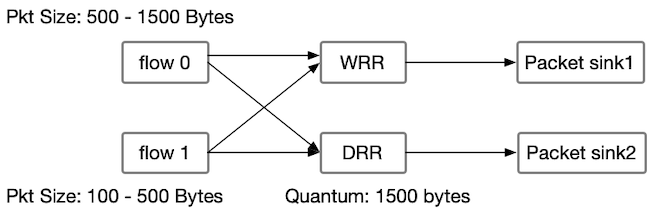The results of the simulation indicate that the WRR scheduler simply alternates between Flow 0 and Flow 1 in a round-robin fashion. However, the DRR scheduler sends more packets from Flow 1 in each iteration, demonstrating the fair and efficient buffer draining achieved by the DRR algorithm.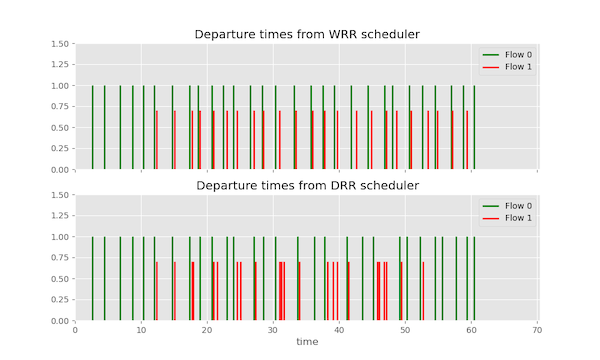# Conclusion

In this post, we explored fairness in buffer allocation and packet scheduling techniques. We identified the limitations of fixed thresholds and introduced dynamic thresholds to adapt buffer sharing based on available capacity, promoting fairness and preventing starvation.

The deficit round-robin (DRR) scheduling algorithm addressed fairness concerns with varying packet sizes, ensuring equitable buffer utilization. Bursty traffic simulations showed the importance of alpha values in improving fairness and reducing packet drops, though complete elimination of drops was not achieved.

While our exploration did not consider packet size distribution and congestion control, it highlighted the significance of fair buffer allocation and efficient scheduling for network performance.

I hope you find this helpful, and if you have any ideas or suggestions, please don’t hesitate to contact me.